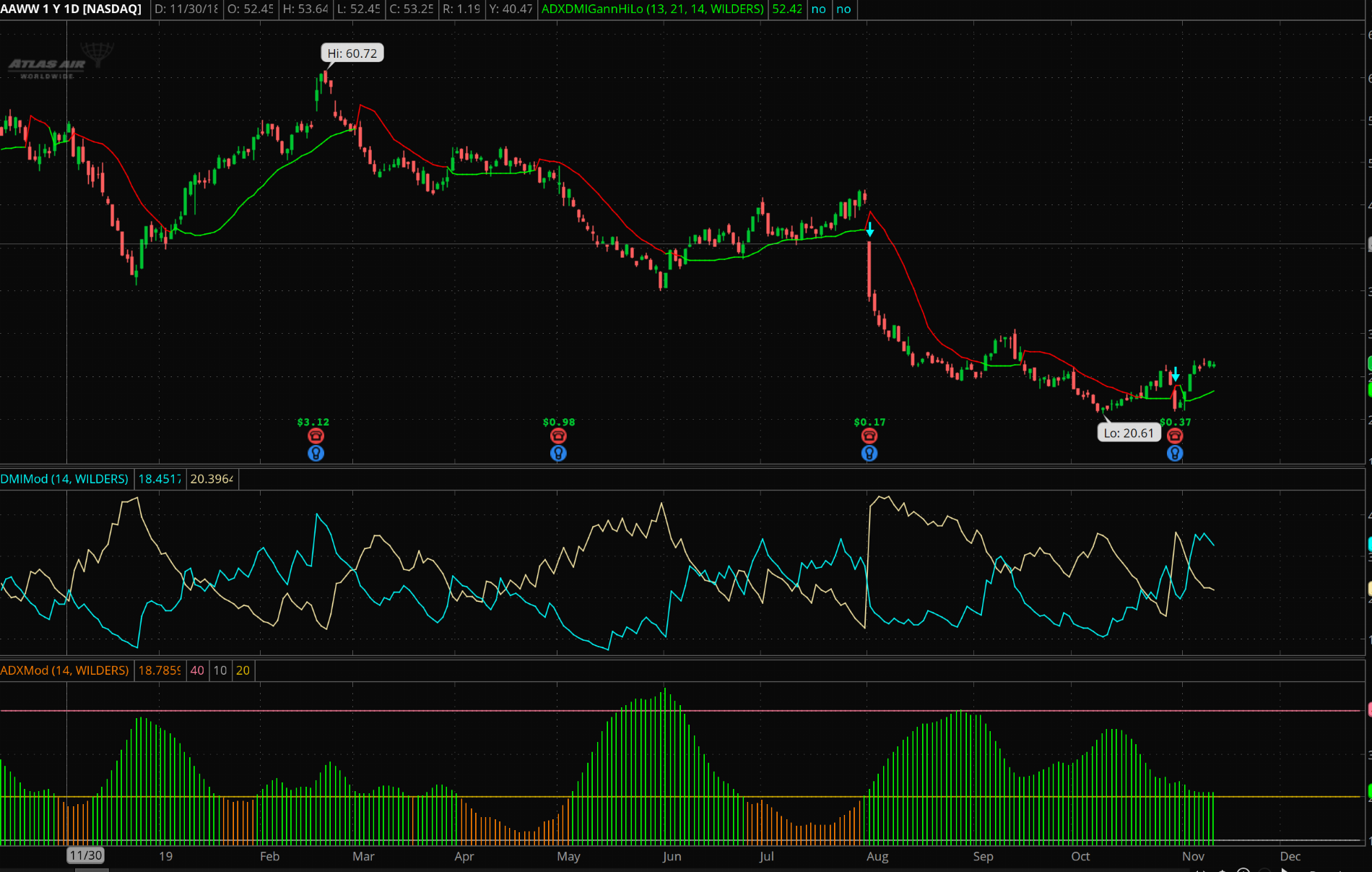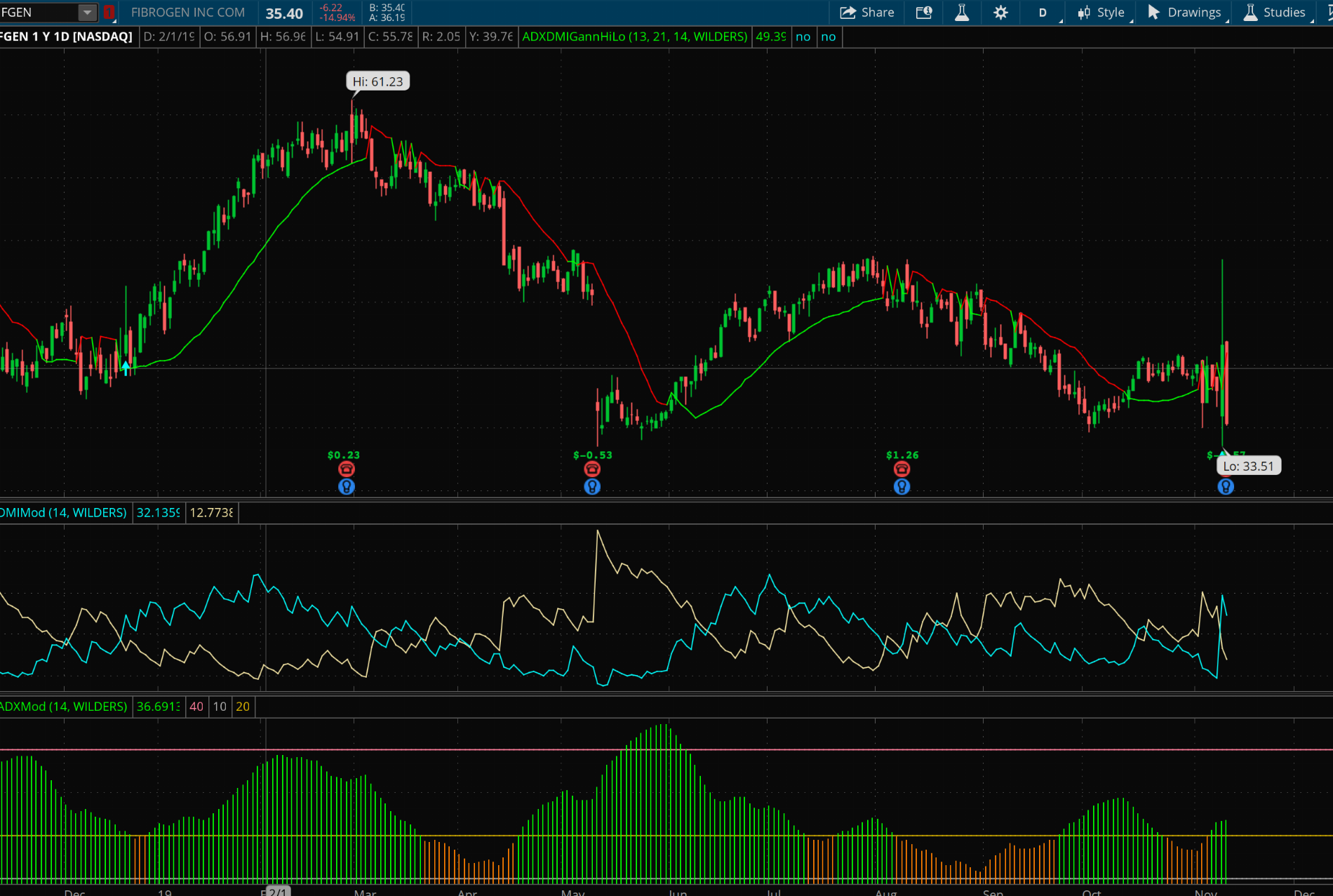# ADX Crossover Indicator System for ThinkorSwim

#### jox51

##### Member
2019 Donor
The below indicator is a replica of the ADX system that Chris Moody coded for TradingView at the link below. Feel free to edit and use as you wish. He uses the Gann Swing Hi Low indicator, I used the PSAR indicator as an alternate. Some images attached below.Code:
``````#
# This study attempts to replicate Chris Moody's ADX System for TOS. All credit goes to him. This study incorporates the GannHiLo study to determine the swing hi's and lows.

# Take a look at the video here where he explains this system.

# This study attempts to catch the beginning of a swing move in a trending underlying. What you want to do is waiting for crossovers of the DI+/DI- respectively while the ADX is beginning to Trend above 20 while the ParabolicSAR is in a supportive Swing High or Low. The buy/sell signals will alert you to this. Use your own discretion.

# When the ADX is over 40, the trend is exhausted and you should be looking at exiting your positions.

# Gann High Low
# Converted by theelderwand

input HPeriod= 13;
input LPeriod= 21;

def HLd = if close > SimpleMovingAvg(high, HPeriod)
then 1
else (if close< SimpleMovingAvg(low, LPeriod)
then -1
else 0);

def HLv = if HLd != 0 then HLd else 0;

plot HiLo = if HLv == -1
then SimpleMovingAvg(high, HPeriod)
else SimpleMovingAvg(low, LPeriod);

HiLo.AssignValueColor(if HLv==-1 then Color.Red else Color.Green);

#Below code is for ADX and DMI confirmation

input length = 14;
input averageType = AverageType.WILDERS;

def hiDiff = high - high;
def loDiff = low - low;

def plusDM = if hiDiff > loDiff and hiDiff > 0 then hiDiff else 0;
def minusDM =  if loDiff > hiDiff and loDiff > 0 then loDiff else 0;

def ATR = MovingAverage(averageType, TrueRange(high, close, low), length);
def "DI+" = 100 * MovingAverage(averageType, plusDM, length) / ATR;
def "DI-" = 100 * MovingAverage(averageType, minusDM, length) / ATR;

def DX = if ("DI+" + "DI-" > 0) then 100 * AbsValue("DI+" - "DI-") / ("DI+" + "DI-") else 0;
def ADX = MovingAverage(averageType, DX, length);

def sell = ADX > 20 && ADX > ADX && "DI-" crosses above "DI+" && HLv==-1;

# Plot Signals
plot sellsignal = sell;
sellsignal.SetPaintingStrategy(PaintingStrategy.BOOLEAN_ARROW_DOWN);
sellsignal.SetDefaultColor(Color.CYAN);
sellsignal.SetLineWeight(1);``````

DMIMod code below https://tos.mx/CUaFybK

Incorporated the GannHiLow study to stay true to the original system.

Thanks @BenTen for pointing me to the GannHiLo study on this site.

#### Attachments

Last edited:
Hi @jox51 -awesome ty! - the buy and sell plots are not plotting not sure if the HLv criteria is valid I changed the buy from <> to != and it started the buy signal, haven't seen a sell condition triggered yet. Have you compared GANN to the PSAR? Something I added to backlog to test. thanks for the conversion.

Here's the built-in ADX crossover script from ThinkorSwim

Code:
``````#wizard text: ADX crosses
#wizard input: crossingType
#wizard input: threshold
#wizard text: Inputs: length:
#wizard input: length

input length = 14;
input crossingType = {default above, below};
input threshold = 20;
input averageType = AverageType.WILDERS;

plot signal = crosses(DMI(length, averageType).ADX, threshold, crossingType == CrossingType.above);

signal.DefineColor("Above", GetColor(0));
signal.DefineColor("Below", GetColor(1));
signal.AssignValueColor(if crossingType == CrossingType.above then signal.color("Above") else signal.color("Below"));

signal.SetPaintingStrategy(if crossingType == CrossingType.above
then PaintingStrategy.BOOLEAN_ARROW_UP
else PaintingStrategy.BOOLEAN_ARROW_DOWN);``````

•dnnfin
I am trying to add a column on my watch list that will tell me how many bars in a row a specified condition has been meet
The condition is DMI+ is greater than or equal to 25 and DMI- is less than or equal to 20 and the ADX is greater than or = to 20
So when this first happens I would like the column to say the number 1 and if it happens for a second candle in a row I would like it to say the number 2 and so on until it doesn't happen than it would drop off my list

Does anyone know of a multi-time frame ADX script? From what I gather because ADX calls up another study (DMI) it isn't as simple as input Period = aggregationPeriod.DAY; or similar.

i.e., I want to be on a 1m chart but have an ADX indicator showing me ADX on 5m.

Thanks!

@hashy Here is a multi-timeframe ADX -
Code:
``````# Multi-Timeframe ADX
# based on DMI indicator code by TD Ameritrade IP Company, Inc. (c) 2008-2020

declare lower;

input length = 14;
input averageType = AverageType.WILDERS;
input aggregation = aggregationperiod.FIVE_MIN;
def h = high(period = aggregation);
def l = low(period = aggregation);
def c = close(period = aggregation);

def hiDiff = h - h;
def loDiff = l - l;

def plusDM = if hiDiff > loDiff and hiDiff > 0 then hiDiff else 0;
def minusDM =  if loDiff > hiDiff and loDiff > 0 then loDiff else 0;

def ATR = MovingAverage(averageType, TrueRange(h, c, l), length);

def "DI+" = 100 * MovingAverage(averageType, plusDM, length) / ATR;
def "DI-" = 100 * MovingAverage(averageType, minusDM, length) / ATR;

def DX = if ("DI+" + "DI-" > 0) then 100 * AbsValue("DI+" - "DI-") / ("DI+" + "DI-") else 0;
plot ADX = MovingAverage(averageType, DX, length);

•zeller427, hashy and BenTen
@MBF Here's the scan code for ADX above 24. Place this in the scanner. I obtained 214 hits on the S&P 500 on a daily aggregation scan

Code:
``````input length = 14;
input averageType = AverageType.WILDERS;
plot scan = ADX > 24;``````

•J007RMC
So I don't own the code. I found it on the web but it is what I was looking for. I also just want to say sorry if this is a stupid question. Is there anyway to add a condition to this code that the ADX has to be above 25?

Code:
``````input length = 14;
input averageType = AverageType.WILDERS;
input threshold = 20;
input lookBack = 90;
def hiDiff = high - high;
def loDiff = low - low;
def plusDM = if hiDiff > loDiff and hiDiff > 0 then hiDiff else 0;
def minusDM = if loDiff > hiDiff and loDiff > 0 then loDiff else 0;
def ATR = MovingAverage(averageType, TrueRange(high, close, low), length);
def diPlus = 100 * MovingAverage(averageType, plusDM, length) / ATR;
def diMinus = 100 * MovingAverage(averageType, minusDM, length) / ATR;
def DX = if (diPlus + diMinus > 0) then 100 * AbsValue(diPlus - diMinus) / (diPlus + diMinus) else 0;
def diPlusCrossesAbove = diPlus < diMinus and diPlus > diMinus;
def diPlusCrossesBelow = diPlus > diMinus and diPlus < diMinus;
# use this scan to check for DI+ crossing above DI-
plot scan = Highest(diPlusCrossesAbove, lookBack) < 1 and diPlusCrossesAbove;
# use this scan to check for DI+ crossing below DI-
#plot scan = Highest(diPlusCrossesBelow, lookBack) < 1 and diPlusCrossesBelow;``````

Last edited by a moderator:
@malinois1983 Here you go... Notice the 1st line and the addition to the end of your plot line...

Ruby:
``````def adx = ADX() >= 25;
input length = 14;
input averageType = AverageType.WILDERS;
input threshold = 20;
input lookBack = 90;
def hiDiff = high - high;
def loDiff = low - low;
def plusDM = if hiDiff > loDiff and hiDiff > 0 then hiDiff else 0;
def minusDM = if loDiff > hiDiff and loDiff > 0 then loDiff else 0;
def ATR = MovingAverage(averageType, TrueRange(high, close, low), length);
def diPlus = 100 * MovingAverage(averageType, plusDM, length) / ATR;
def diMinus = 100 * MovingAverage(averageType, minusDM, length) / ATR;
def DX = if (diPlus + diMinus > 0) then 100 * AbsValue(diPlus - diMinus) / (diPlus + diMinus) else 0;
def diPlusCrossesAbove = diPlus < diMinus and diPlus > diMinus;
def diPlusCrossesBelow = diPlus > diMinus and diPlus < diMinus;
# use this scan to check for DI+ crossing above DI-
plot scan = Highest(diPlusCrossesAbove, lookBack) < 1 and diPlusCrossesAbove;
# use this scan to check for DI+ crossing below DI-
#plot scan = Highest(diPlusCrossesBelow, lookBack) < 1 and diPlusCrossesBelow and adx;``````

### Not the exact question you're looking for?

87k+ Posts
348 Online## The Market Trading Game Changer

Join 2,500+ subscribers inside the useThinkScript VIP Membership Club
• Exclusive indicators
• Proven strategies & setups
• Private Discord community
• Exclusive members-only content
• 1 full year of unlimited support

What is useThinkScript?

useThinkScript is the #1 community of stock market investors using indicators and other tools to power their trading strategies. Traders of all skill levels use our forums to learn about scripting and indicators, help each other, and discover new ways to gain an edge in the markets.

How do I get started?

We get it. Our forum can be intimidating, if not overwhelming. With thousands of topics, tens of thousands of posts, our community has created an incredibly deep knowledge base for stock traders. No one can ever exhaust every resource provided on our site.

If you are new, or just looking for guidance, here are some helpful links to get you started.

What are the benefits of VIP Membership?